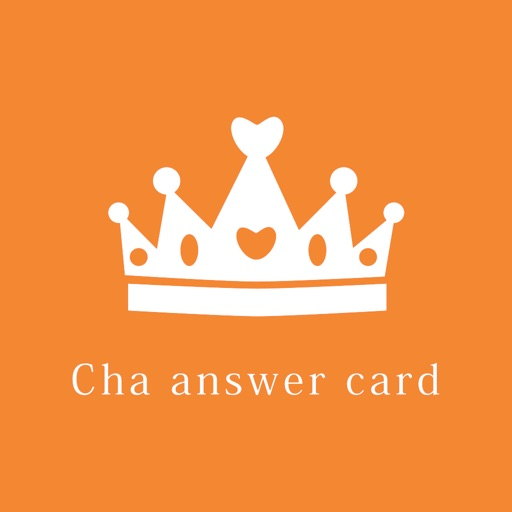## An answer card application on mathematical calculations to improve the speed of mathematical calculations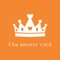by 建卫 廖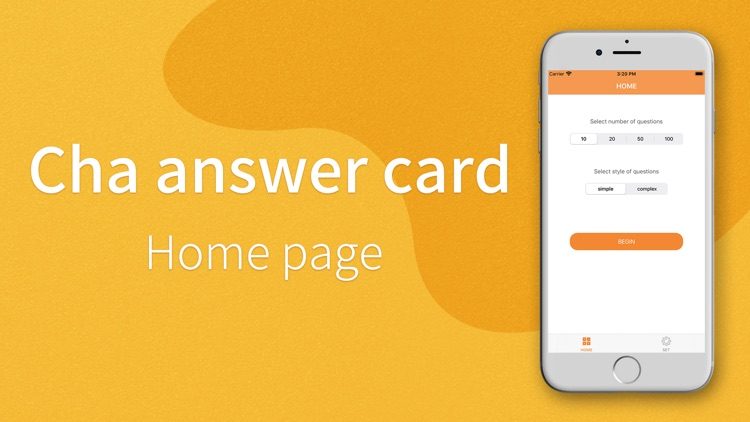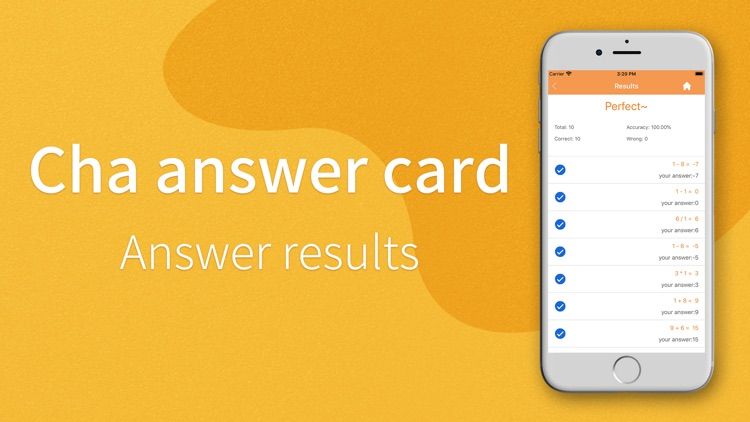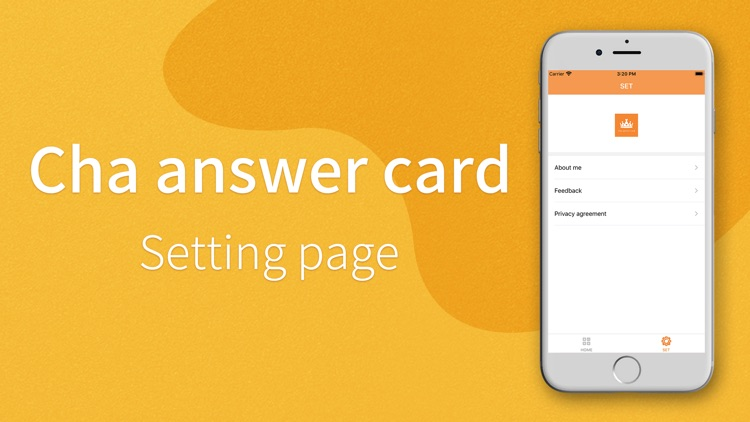An answer card application on mathematical calculations to improve the speed of mathematical calculations.Version
1.0
Rating
NA
Size
19Mb
Genre
Education
Last updated
June 20, 2020
Release date
June 20, 2020